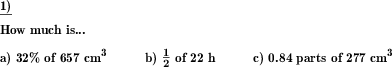Custom math worksheets at your fingertips# Details for problem "Fractions of units of measurement"

Quickname: 3400

Elementary School, Primary School, Junior High School, Middle School, High School.

## Summary

Identify fractions of area, price, weight, length given in different forms: 1/8, 20%, 0.3.

## Example## Description

Fractions of sizes shall be indicated. The fractions can be specified as fractions, decimal fractions or percentages. The type of the fraction specified can be chosen to be the same for all problems or mixed. The same applies to the unit of measurement. These physical quantities are available:

• Money (Euro, Cent)
• Money (dollars, cents)
• Length
• Volume
• Time
• Weight
• Area

Either a specific physical quantity can be chosen for all problems, or a random one will be selected for each problem.

With the formation of the fractions it is possible to leave the unit step, such as 10% of 1 l = 100ml. As a simplification, this can be excluded, so that only tasks in the style of 50% of 500ml=250ml occur, so that the unit of measurement does not play a defining role for the problem.

The number of problems can also be specified.

Download free printable worksheets for this math problem here. The worksheet contains the problems only, the solution sheet includes the answers. Just click on the respective link.

•Worksheet 1Solution sheet with answers
•Worksheet 2Solution sheet with answers
•Worksheet 3Solution sheet with answers

If you can not see the solution sheets for download, they may be filtered out by an ad blocker that you may have installed. If this is the case, please allow ads for this page and reload the page. The solution sheets will then reappear.

• Do these sample worksheets do not really fit?
• Do you need more math worksheets, with a different level of difficulty?
• Would you like to combine different problems on a worksheet and adjust them to your needs?
• As a teacher, you can put together your own worksheets using the automatically generated math problems provided.
With a free initial credit, you can start creating your own math worksheets in a few minutes.

You can try it for free! Register here, to create custom worksheets now!

## Customization options for this problem

Parameter
Possible values
Number of problems
1, 2, 3, 4, 5, 6, 7, 8, 9, 10
Type of specification
mixed, decimal, fraction, percent
Unit
mixed, Money (USD), Money (EUR), Weight, Time, Volume, Area, Length
More than one unit step
Yes, No